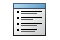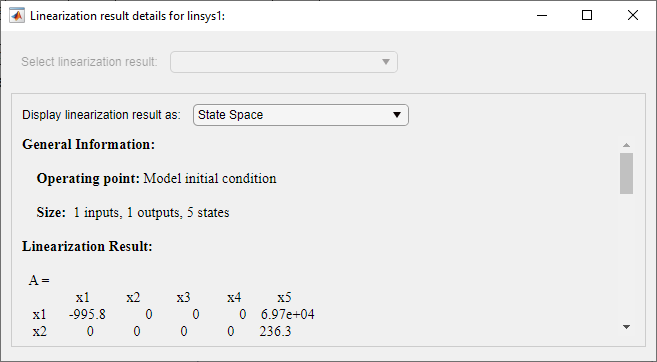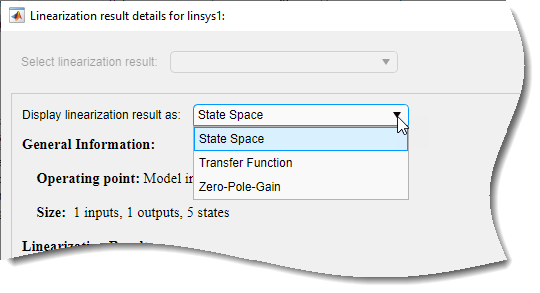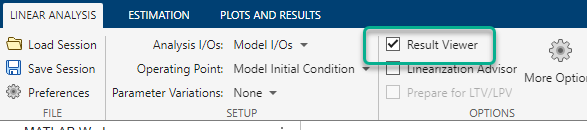View Linearized Model Equations Using Model Linearizer

When you linearize a Simulink® model using the Model Linearizer, the software generates state-space equations for the resulting linear model. To view the linearized model equations:

1. In the Data Browser, in the Linear Analysis Workspace, select the linear model you want to view.

2. On the Plots and Results tab, clickResult Viewer.In the Linearization result details dialog box, the software displays:

• General information about the linearization, including the operating point and the number of inputs, outputs, and states.

• State-space matrices for the linearized model.

• Lists of the state, input, and output names. To highlight a state, input, or output in the Simulink model, click the corresponding name.

To display the system using either zero-pole-gain or transfer function equations, in the Display linearization result as drop-down list, select a format.You can automatically open the Linearization result details dialog box when you linearize your model. To do so, on the Linear Analysis tab, select Result Viewer before you linearize the model.